# MATCH Worksheet Function in Excel, VBA, & Google Sheets

This Excel Tutorial demonstrates how to use the Excel MATCH Function in Excel to find a value, with formula examples.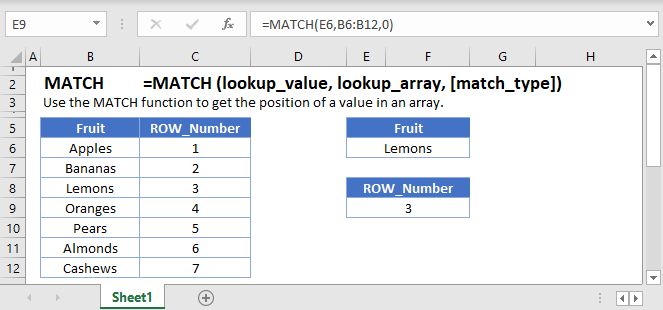## MATCH Function overview

The MATCH Function Searches for an item in a list and returns a number representing it’s position in the list.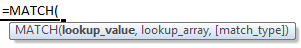(Notice how the formula input appear)

### Match Function syntax and Argument

lookup_value – The value you want to search for.

lookup_array – An array of data either one column wide or one row high that you want to search in.

match_type – 0,-1 or 1 specifies what to do if an exact match is not found. 0 returns an error. -1 returns the nearest match that is greater than the lookup_value. 1 returns the nearest match that is less than the lookup_value.

### What is the MATCH function?

In simple terms, the MATCH function can search a range/array of items and return the relative position of the searched word. It is often used in conjunction with the INDEX function, since INDEX needs a relative position to return a result.

### Exact match

You will often come across large lists of data and you need to be able to search for a specific item. We’ll use a small example with some fruit. First, we’ll look for an exact match. Here’s the layout of our data. We want to search for the word in cell D1.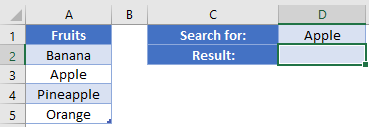In D2, our formula is: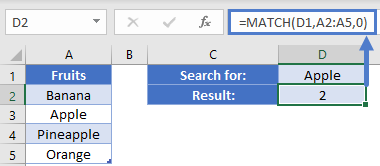Take note that we needed to specify either 0 or False as the last argument to indicate that we want an exact match. The result from this function is 2, because “Apple” is the 2nd item in our range.

### Sorted list

Let’s see how the MATCH function works with a non-exact match. Here we have a list of items. NOTE: The items have been sorted in ascending order.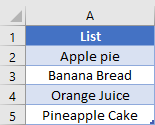In D1, we’ve said that we want to look for the word “Orange”. The formula in D2 is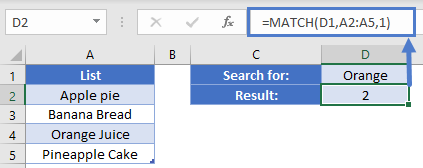Our formula gives a result of 2 even though “Orange Juice” is in the 3rd cell. Because we were searching for a closest match, the function will find either our exact word, or the next smallest item. With text strings, this happens to be the word just before “Orange Juice”, and so we got a result of 2.

This ability to find next smallest may be easier to understand using a number search. Consider this layout, where we’ve only listed items by 10. If we search for a value of 34 using our formula, you can see that the result is 3.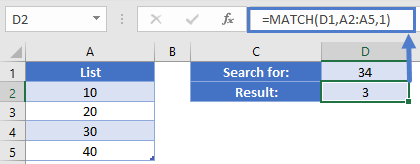This  can work well when you are dealing with “buckets”, and you just want to be able to find out which group a value belongs in.

### Descending list

In our previous example, what if you wanted the value of 34 to be placed in the larger group? In our next example, let’s say that we have a list of difference sized shipping containers and we need to know which one to use. Since we need to make sure we have enough room or more, we’ll use the MATCH function with the last argument set to -1. Let’s look at this example: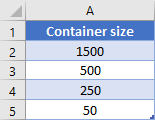In this case, we need to find out which container will fit our size of 495. The formula in D2 is: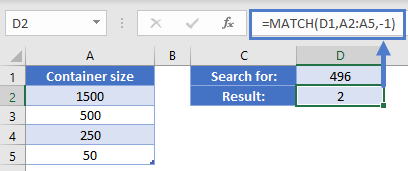The result of this formula is 2, indicating that we need to use the 2nd item from the list (the 500) to fit our needs.

### Wildcard match

The MATCH function also supports the use of wildcards like “*” and “?”. Let’s go back to our list of various food items. In this case, we’ve changed our search term in D1 to be “Orange*”.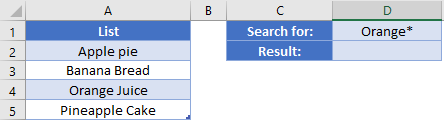Our formula in D2 is: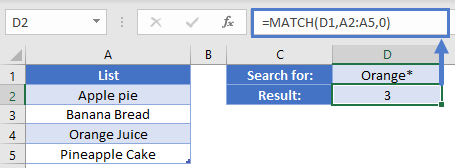Note that we need to switch the MATCH type back to exact match. Even though the items are mixed up and our search term did not have the full text match, our formula was able to give the correct result of 3.

### Using MATCH with INDEX

Returning the relative position of an item is nice, but it’s usually not that helpful to human users. We usually want to know the corresponding value for an item. This is where INDEX can enter the scene. As INDEX needs a numerical position for row and/or column, we can use the MATCH function to tell the INDEX which item we want. Consider the following layout where we need to be able to lookup prices for our items.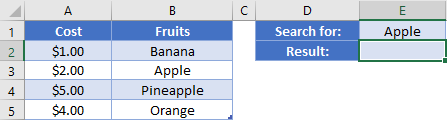We are searching for “Apple”. In E2, our formula is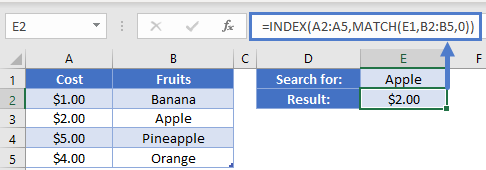The MATCH function is going to search B2:B5 for our exact phrase, “Apple”. It will find this in the 2nd item and return the value of 2. The INDEX then takes this and will give us the 2nd value from the A2:A5 range. The 2nd item is in cell A3, the \$2.00. This is discussed more in <link to another article>

The MATCH Function works exactly the same in Google Sheets as in Excel: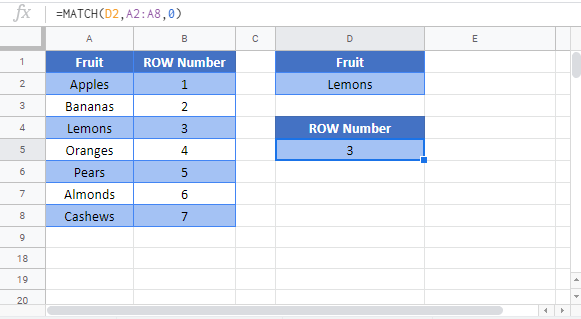<

Use the MATCH Function to find the numerical postion of a value within a range of values. The range must be either a subset of a single range or a single row.

The MATCH Function is most frequently used with the INDEX Function.

## MATCH Examples in VBA

You can also use the MATCH function in VBA. Type:

For the function arguments (lookup_value, etc.), you can either enter them directly into the function, or define variables to use instead.

Assuming we have the following values in our Excel sheet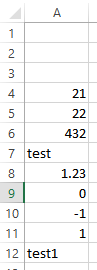executing the following code

Will return the following

because the string “test1” is the ninth element in the range of values to be searched.

We can also search for content in our worksheet instead of directly entering values in the VBA  code: The following statement will search Column A for the whatever value is entered in cell C3